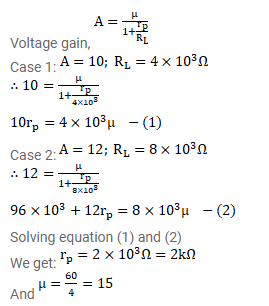# The gain factor of an amplifier is increased from 10 to 12

Question:

The gain factor of an amplifier is increased from 10 to 12 as the load resistance is changed from $4 \mathrm{k} \Omega$ to $8 \mathrm{k} \Omega$. Calculate (a) the amplification factor and (b) the plate resistance.

Solution: